### Calculate force given work and displacement.

#### Example question:

How much force is required to do 110 J of work over a displacement of 12 m?

#### Given:

work: W = 110 J

displacement: d = 12 m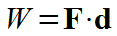Starting formula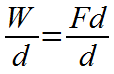Algebra: Divide each side by d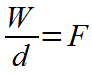Algebra: Cancel d's on right side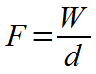Algebra: Switch left and right sides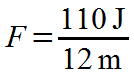Plug in numbers with units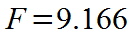Divide: 110/12 = 9.166....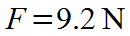Final result with significant figures and unit